xCarbonic acidEncyclopedia
Carbonic acid is also an archaic name for carbon dioxide
Carbon dioxide
Carbon dioxide is a naturally occurring chemical compound composed of two oxygen atoms covalently bonded to a single carbon atom...

Carbonic acid is the inorganic compound
Inorganic compound
Inorganic compounds have traditionally been considered to be of inanimate, non-biological origin. In contrast, organic compounds have an explicit biological origin. However, over the past century, the classification of inorganic vs organic compounds has become less important to scientists,...

with the formula H2CO3 (equivalently OC(OH)2). It is also a name sometimes given to solution
Solution
In chemistry, a solution is a homogeneous mixture composed of only one phase. In such a mixture, a solute is dissolved in another substance, known as a solvent. The solvent does the dissolving.- Types of solutions :...

s of carbon dioxide
Carbon dioxide
Carbon dioxide is a naturally occurring chemical compound composed of two oxygen atoms covalently bonded to a single carbon atom...

in water, because such solutions contain small amounts of H2CO3. Carbonic acid forms two kinds of salts, the carbonate
Carbonate
In chemistry, a carbonate is a salt of carbonic acid, characterized by the presence of the carbonate ion, . The name may also mean an ester of carbonic acid, an organic compound containing the carbonate group C2....

s and the bicarbonate
Bicarbonate
In inorganic chemistry, bicarbonate is an intermediate form in the deprotonation of carbonic acid...

s. It is a weak acid
Weak acid
A weak acid is an acid that dissociates incompletely. It does not release all of its hydrogens in a solution, donating only a partial amount of its protons to the solution...

.

## Chemical equilibrium

When carbon dioxide dissolves in water it exists in chemical equilibrium
Chemical equilibrium
In a chemical reaction, chemical equilibrium is the state in which the concentrations of the reactants and products have not yet changed with time. It occurs only in reversible reactions, and not in irreversible reactions. Usually, this state results when the forward reaction proceeds at the same...

producing carbonic acid:
CO2 + H2O H2CO3

The hydration
Hydrate
Hydrate is a term used in inorganic chemistry and organic chemistry to indicate that a substance contains water. The chemical state of the water varies widely between hydrates, some of which were so labeled before their chemical structure was understood....

equilibrium constant at 25 °C is called Kh, which in the case of carbonic acid is [H2CO3]/[CO2] = 1.70×10−3: hence, the majority of the carbon dioxide is not converted into carbonic acid, remaining as CO2 molecules. In the absence of a catalyst, the equilibrium is reached quite slowly. The rate constants are 0.039 L/mol−1*s−1 for the forward reaction (CO2 + H2O → H2CO3) and 23 s−1 for the reverse reaction (H2CO3 → CO2 + H2O).
Carbonic acid is used in the making of soft drinks, inexpensive and artificially carbonated sparkling wines, and other bubbly drinks. The addition of two equivalents of water to CO2 would give orthocarbonic acid
Orthocarbonic acid
Orthocarbonic acid or methanetetraol is the name given to a hypothetical compound with the chemical formula H4CO4 or C4. Its molecular structure consists of a single carbon atom bonded to four hydroxyl groups. It would be therefore a fourfold alcohol...

, C(OH)4, which exists only in minute amounts in aqueous solution.

Addition of base to an excess of carbonic acid gives bicarbonate
Bicarbonate
In inorganic chemistry, bicarbonate is an intermediate form in the deprotonation of carbonic acid...

. With excess base, carbonic acid reacts to give carbonate
Carbonate
In chemistry, a carbonate is a salt of carbonic acid, characterized by the presence of the carbonate ion, . The name may also mean an ester of carbonic acid, an organic compound containing the carbonate group C2....

salts.

## Role of carbonic acid in blood

Carbonic acid is an intermediate step in the transport of CO2 out of the body via respiratory gas exchange
Gas exchange
Gas exchange is a process in biology where gases contained in an organism and atmosphere transfer or exchange. In human gas-exchange, gases contained in the blood of human bodies exchange with gases contained in the atmosphere. Human gas-exchange occurs in the lungs...

. The hydration reaction of CO2 is generally very slow in the absence of a catalyst, but red blood cells contain carbonic anhydrase
Carbonic anhydrase
The carbonic anhydrases form a family of enzymes that catalyze the rapid interconversion of carbon dioxide and water to bicarbonate and protons , a reversible reaction that occurs rather slowly in the absence of a catalyst...

, which both increases the reaction rate and dissociates a hydrogen ion (H+) from the resulting carbonic acid, leaving bicarbonate
Bicarbonate
In inorganic chemistry, bicarbonate is an intermediate form in the deprotonation of carbonic acid...

(HCO3-) dissolved in the blood plasma
Blood plasma
Blood plasma is the straw-colored liquid component of blood in which the blood cells in whole blood are normally suspended. It makes up about 55% of the total blood volume. It is the intravascular fluid part of extracellular fluid...

. This catalysed reaction is reversed in the lungs, where it converts the bicarbonate back into CO2 and allows it to be expelled. This equilibration plays an important role as a buffer
Buffer solution
A buffer solution is an aqueous solution consisting of a mixture of a weak acid and its conjugate base or a weak base and its conjugate acid. It has the property that the pH of the solution changes very little when a small amount of strong acid or base is added to it. Buffer solutions are used as a...

in mammalian blood.

## Role of carbonic acid in ocean chemistry

The oceans of the world have absorbed almost half of the CO2 emitted by humans from the burning of fossil fuels.  The extra dissolved carbon dioxide has caused the ocean's average surface pH to shift by about 0.1 unit from pre-industrial levels. This process is known as ocean acidification
Ocean acidification
Ocean acidification is the name given to the ongoing decrease in the pH and increase in acidity of the Earth's oceans, caused by the uptake of anthropogenic carbon dioxide from the atmosphere....

.

## Acidity of carbonic acid

Carbonic acid is diprotic
Diprotic acid
A diprotic acid is an acid such as H2SO4 that contains within its molecular structure two hydrogen atoms per molecule capable of dissociating in water. The complete dissociation of diprotic acids is of the same form as sulfuric acid:The dissociation does not happen all at once due to the two...

: it has two protons, which may dissociate from the parent molecule. Thus there are two dissociation constants
Acid dissociation constant
An acid dissociation constant, Ka, is a quantitative measure of the strength of an acid in solution. It is the equilibrium constant for a chemical reaction known as dissociation in the context of acid-base reactions...

, the first one for the dissociation into the bicarbonate
Bicarbonate
In inorganic chemistry, bicarbonate is an intermediate form in the deprotonation of carbonic acid...

(also called hydrogen carbonate) ion HCO3:
H2CO3 HCO3 + H+
Ka1 = 4.45×10−7 ; pKa1 = 6.352 at 25 °C.

With a pKa1 of 6.352, carbonic acid H2CO3 is almost 10x weaker acid than acetic acid
Acetic acid
Acetic acid is an organic compound with the chemical formula CH3CO2H . It is a colourless liquid that when undiluted is also called glacial acetic acid. Acetic acid is the main component of vinegar , and has a distinctive sour taste and pungent smell...

.

The second for the dissociation of the bicarbonate
Bicarbonate
In inorganic chemistry, bicarbonate is an intermediate form in the deprotonation of carbonic acid...

ion into the carbonate
Carbonate
In chemistry, a carbonate is a salt of carbonic acid, characterized by the presence of the carbonate ion, . The name may also mean an ester of carbonic acid, an organic compound containing the carbonate group C2....

ion CO32−:
HCO3 CO32− + H+
Ka2 = 4.69×10−11 ; pKa2 = 10.329 at 25 °C and Ionic Strength = 0.0.

Care must be taken when quoting and using the first dissociation constant of carbonic acid. In aqueous solution carbonic acid only exists in equilibrium with carbon dioxide, and the concentration of H2CO3 is much lower than the dissolved CO2 concentration. Since it is not possible to distinguish between H2CO3 and dissolved CO2 (referred to as CO2(aq)) by conventional methods, H2CO3* is used to represent the two species when writing the aqueous chemical equilibrium equation. The equation may be rewritten as follows (cf. sulfurous acid
Sulfurous acid
Sulfurous acid is the chemical compound with the formula H2SO3. There is no evidence that sulfurous acid exists in solution, but the molecule has been detected in the gas phase...

):
H2CO3* HCO3 + H+
Ka = 4.6×10−7(General Chemistry: An Integrated Approach Third Edition); pKa = 6.352 at 25 °C and Ionic Strength = 0.0.(NIST CRITICAL Database)

Whereas this pKa is quoted as the dissociation constant of carbonic acid, it is ambiguous: it might better be referred to as the acidity constant of dissolved carbon dioxide, as it is particularly useful for calculating the pH
PH
In chemistry, pH is a measure of the acidity or basicity of an aqueous solution. Pure water is said to be neutral, with a pH close to 7.0 at . Solutions with a pH less than 7 are said to be acidic and solutions with a pH greater than 7 are basic or alkaline...

of CO2-containing solutions.

### pH and composition of carbonic acid solutions

At a given temperature, the composition of a pure carbonic acid solution (or of a pure CO2 solution) is completely determined by the partial pressure
Partial pressure
In a mixture of ideal gases, each gas has a partial pressure which is the pressure which the gas would have if it alone occupied the volume. The total pressure of a gas mixture is the sum of the partial pressures of each individual gas in the mixture....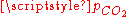of carbon dioxide above the solution. To calculate this composition, account must be taken of the above equilibria between the three different carbonate forms (H2CO3, HCO3 and CO32−) as well as of the hydration equilibrium between dissolved CO2 and H2CO3 with constant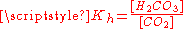(see above) and of the following equilibrium between the dissolved CO2 and the gaseous CO2 above the solution:
CO2(gas) CO2(dissolved) with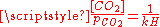where kH=29.76 atm/(mol/L) at 25°C (Henry constant
Henry's law
In physics, Henry's law is one of the gas laws formulated by William Henry in 1803. It states that:An equivalent way of stating the law is that the solubility of a gas in a liquid at a particular temperature is proportional to the pressure of that gas above the liquid...

)

The corresponding equilibrium equations together with the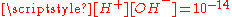relation and the charge neutrality condition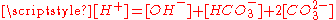result in six equations for the six unknowns [CO2], [H2CO3], [H+], [OH], [HCO3] and [CO32−], showing that the composition of the solution is fully determined by. The equation obtained for [H+] is a cubic whose numerical solution yields the following values for the pH and the different species concentrations:(atm)
pH [CO2]
(mol/L)
[H2CO3]
(mol/L)
[HCO3]
(mol/L)
[CO32−]
(mol/L)
10−8 7.00 3.36 × 10−10 5.71 × 10−13 1.42 × 10−9 7.90 × 10−13
10−7 6.94 3.36 × 10−9 5.71 × 10−12 5.90 × 10−9 1.90 × 10−12
10−6 6.81 3.36 × 10−8 5.71 × 10−11 9.16 × 10−8 3.30 × 10−11
10−5 6.42 3.36 × 10−7 5.71 × 10−9 3.78 × 10−7 4.53 × 10−11
10−4 5.92 3.36 × 10−6 5.71 × 10−9 1.19 × 10−6 5.57 × 10−11
}3.5 × 10−4
| 5.65
|style="color:#000"| 1.18 × 10−5
|style="color:#666"| 2.00 × 10−8
|style="color:#666"| 2.23 × 10−6
|style="color:#BBB"| 5.60 × 10−11
|-
|scope="row"| 10−3
| 5.42
|style="color:#000"| 3.36 × 10−5
|style="color:#666"| 5.71 × 10−8
|style="color:#666"| 3.78 × 10−6
|style="color:#BBB"| 5.61 × 10−11
|-
|scope="row"| 10−2
| 4.92
|style="color:#000"| 3.36 × 10−4
|style="color:#666"| 5.71 × 10−7
|style="color:#666"| 1.19 × 10−5
|style="color:#BBB"| 5.61 × 10−11
|-
|scope="row"| 10−1
| 4.42
|style="color:#000"| 3.36 × 10−3
|style="color:#666"| 5.71 × 10−6
|style="color:#666"| 3.78 × 10−5
|style="color:#BBB"| 5.61 × 10−11
|-
|scope="row"| 100
| 3.92
|style="color:#000"| 3.36 × 10−2
|style="color:#666"| 5.71 × 10−5
|style="color:#666"| 1.20 × 10−4
|style="color:#BBB"| 5.61 × 10−11
|-style="background-color:#AFF"
|scope="row"| 2.5 × 100
| 3.72
|style="color:#000"| 8.40 × 10−2
|style="color:#666"| 1.43 × 10−4
|style="color:#666"| 1.89 × 10−4
|style="color:#BBB"| 5.61 × 10−11
|-
|scope="row"| 101
| 3.42
|style="color:#000"| 3.36 × 10−1
|style="color:#000"| 5.71 × 10−4
|style="color:#666"| 3.78 × 10−4
|style="color:#BBB"| 5.61 × 10−11
|}
• We see that in the total range of pressure, the pH is always largely lower than pKa2 so that the CO32− concentration is always negligible with respect to HCO3 concentration. In fact CO32− plays no quantitative role in the present calculation (see remark below).
• For vanishing, the pH is close to the one of pure water (pH = 7) and the dissolved carbon is essentially in the HCO3 form.
• For normal atmospheric conditions (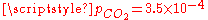atm), we get a slightly acid solution (pH = 5.7) and the dissolved carbon is now essentially in the CO2 form. From this pressure on, [OH] becomes also negligible so that the ionized part of the solution is now an equimolar mixture of H+ and HCO3.
• For a CO2 pressure typical of the one in soda drink bottles (~ 2.5 atm), we get a relatively acid medium (pH = 3.7) with a high concentration of dissolved CO2. These features contribute to the sour and sparkling taste of these drinks.
• Between 2.5 and 10 atm, the pH crosses the pKa1 value (3.60) giving a dominant H2CO3 concentration (with respect to HCO3) at high pressures.

Remark
As noted above, [CO32−] may be neglected for this specific problem, resulting in the following very precise analytical expression for [H+]: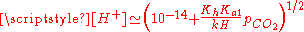## Spectroscopic studies of carbonic acid

Theoretical calculations show that the presence of even a single molecule of water causes carbonic acid to revert to carbon dioxide and water. In the absence of water, the dissociation of gaseous carbonic acid is predicted to be very slow, with a half-life
Half-life
Half-life, abbreviated t½, is the period of time it takes for the amount of a substance undergoing decay to decrease by half. The name was originally used to describe a characteristic of unstable atoms , but it may apply to any quantity which follows a set-rate decay.The original term, dating to...

of 180,000 years.

It has long been recognized that pure carbonic acid cannot be obtained at room temperatures (about 20 °C or about 70 °F). It can be generated by exposing a frozen mixture of water and carbon dioxide to high-energy radiation, and then warming to remove the excess water. The carbonic acid that remained was characterized by infrared spectroscopy. The fact that the carbonic acid was prepared by irradiating a solid H2O + CO2 mixture may suggest that H2CO3 might be found in outer space, where frozen ices of H2O and CO2 are common, as are cosmic rays and ultraviolet light, to help them react. The same carbonic acid polymorph (denoted beta-carbonic acid) was prepared by heating alternating layers of glassy aqueous solutions of bicarbonate and acid in vacuo, which causes protonation of bicarbonate, followed by removal of the solvent. Alpha-carbonic acid was prepared by the same technique using methanol rather than water as a solvent.